# GSEB Solutions Class 11 Physics Chapter 6 Work, Energy and Power

Gujarat Board GSEB Textbook Solutions Class 11 Physics Chapter 6 Work, Energy and Power Textbook Questions and Answers.

## Gujarat Board Textbook Solutions Class 11 Physics Chapter 6 Work, Energy and Power

### GSEB Class 11 Physics Work, Energy and Power Text Book Questions and Answers

Question 1.
The sign of wrk done by a force on a body is important to understand. State carefully if the following quantities are positive or negative:

1. Work done by a man in lifting a bucket out of a well by means of a rope tied to the bucket.
2. Work done by gravitational force in the above case.
3. Work done by friction on a body sliding down an inclined plane.
4. Work done by an applied force on a body moving on a rough horizontal plane with uniform velocity.
5. Work done by the resistive force of air on a vibrating pendulum in bringing it to rest.

We know that the work done,is given by W = F.S = FS cos θ,
where θ = smaller angle between F (force) and displacement S.

1. To lift the bucket, force equal to the weight of the bucket has to be applied vertically upwards and the bucket moves along the same direction, thus θ = 0, so W = FS i.e. positive.

2. Here as the bucket moves in a direction opposite to the gravitational force which always acts vertically downwards.
∴ θ = 180°,
so W = FScosl80° = F\$(-1) = -FS
i.e. W = negative.

3. Work done by friction on a body sliding down an inclined plane is negative as friction opposes the relative motion,
thus θ = 180° ∴ W = -FS.

4. As the body moves in the same direction in which the force is applied, so θ = 0, thus W = FS i.e. it is positive.

5. Work done is negative as the direction of the resistive force of air on the vibrating pendulum is opposite to the direction of displacement (i.e. motion) of the bob.Question 2.
A body of mass 2 kg initially at rest moves under the action of an applied horizontal force of 7 N on a table with coefficient of kinetic friction = 0.1. Compute the

1. work done by the applied force is 10s.
2. work done by friction in 10s.
3. work done by the net force on the body in 10s.
4. change in kinetic energy of the body in 10s and interpret your results.

Here, m = 2 kg, F = 7 N, initial velocity, u = 0,
Coefficient of kinetic friction, µk = 0.1, time t = 10s
Force of friction, µf = R = µmg
= 0.1 × 2 × 9.8
= 1.96 N
∴ Net force F’ = F – f
= (7 – 1.96); N = 5.04N
∴ Net acceleration with which the body moves,
a = $$\frac{F}{m}$$ = $$\frac{5.04}{2}$$ = 2.52 ms-2
If S be the distance covered by the body in 10s, then
S = ut + $$\frac{F}{m}$$ at2
= 0 × 10 + $$\frac{1}{2}$$ × 2.52 × (10)2
= 1.26 × 100m
S = 126 m
∴ S = 126 m

1. Work done by the applied force,
W = FS = 7 × 126
= 882 J

2. Work done by the force of friction,
W2 = – fS
= – 1.96 × 126
= -246.96 J
= – 247 J

3. Work done by the net force,
W3 = FS
= 5.04 × 126
= 635.04 J

Aliter:
W3 = W1 – W2
= (882 – 246.96) J
= 635.04 J

4. According to work – energy theorem,
Change in kinetic energy = work done
or change in K.E. = 635.04 J

Aliter:
v = final velocity after 10s
= u + at
0 + 2.52 × 10
= 25.2 ms-1
∴ (K.E.)f = $$\frac{1}{2}$$mv2 = $$\frac{1}{2}$$ × 2 × (25.2)2
= 635.04 J
also (K.E.)i = $$\frac{1}{2}$$mu2 = $$\frac{1}{2}$$ × 2 × (0)2 = 0
∴ change in K.E. = (K.E.)f – (K.E.)i
= 635.04 – 0 = 635.04 J
i.e. change in K.E. of the body = work done by the net force on the body.Question 3.
Given below are examples of some potential energy) functions in one dimension. The total energy of the particle is indicated by a cross on the ordinate axis. In each case, specify the regions, if any, in which the particle cannot be found for the given energy. Also, indicate the minimum total energy the particle must have in each case. Think of simple physical contexts for which these potential energy shapes are relevant?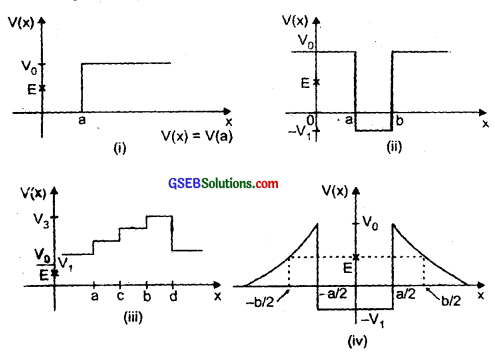We know that the total energy of the body is given by
E = K.E. + P.E.
or K.E. = E – P.E.= $$\frac{1}{2}$$mv2
So K.E. of the body can never be negative. Thus, P.E. cannot be greater than E.

1. In the region between x = 0 and x = a, P.E. is zero, so K.E. is positive. In the region x > a, the P.E. (V0) has a value greater than E. So K.E. will be negative in this region. Thus, the particle can’t be present in the region x > a. The minimum total energy that the particle can have in this
case is zero.

2. In region x < a and x > b, the P.E. is vo which is greater than the total energy of the particle. So K.E. will be negative in this region. Thus the particle cannot he present in the region x < a and x > b. In the region between x > a and x < b, the P.E. is negative. This means that there is a positive value of K.E. so the particle can be present in the region between x > a and x < b. The minimum total energy that the particle can have in this case is – V1.

3. Here in all the regions i. e. – ∞ < x < a, a < x < c, c < x < b, b < x < d and d < x < the P.E. is greater than the total energy. So, the particle cannot be present in the region – ∞ < x < ∞. The minimum total energy that the particle can have in this case is V1.

4. In this case, the P.E. of the particle is move than the total energy (E) in the regions – $$\frac{b}{2}$$ < x < – $$\frac{a}{2}$$ and $$\frac{a}{2}$$ < x < $$\frac{b}{2}$$.
So, K.E. of the particle will be negative in this regions, thus it will not be present m the regions – $$\frac{b}{2}$$, x < – $$\frac{a}{2}$$ and $$\frac{a}{2}$$ < x < $$\frac{b}{2}$$. The minimum total energy that the particle can have in this case is – V1.

Question 4.
The potential energy function for a particle executing linear simple harmonic motion is given by V(x) = $$\frac{a}{2}$$ kx2 where k is the force constant of the oscillator. For k = 0.5 Nm-1, the graph V(x) versus x is shown in the figure given below. Show that a particle of total energy 1 J moving under this potential must turn back when it reaches x = ± 2m?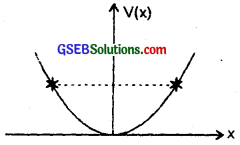At any instant, the total energy (E) of an oscillator is partially K.E. and partially P.E., thus E can be expressed as the sum of K.E. and P.E. i.e.
E = K.E. + P.E.
or 1 = $$\frac{1}{2}$$ mu2 + $$\frac{1}{2}$$ kx2 ……………………. (i)
when m = mass of the particle,
k = force constant
= 0.5 Nm-1 (given)
x = displacement
u = velocity of the particle
E = 1 J (given)
An oscillating particle turns back at the instant where its velocity or speed becomes zero i.e. u = 0 ……………………….. (ii)
and total energy is equal to the P.E. from
∴ (i) and (ii), we get
1 = 0 + $$\frac{1}{2}$$ kx2
x2 = $$\frac{2×1}{k}$$ = $$\frac{2×1}{0.5}$$ = 2 × 1 × 2 = 4
x = ± 2m, Hence proved.Question 5.
(a) The casing of a rocket in flight burns up due to friction. At whose expense is the heat energy required for burning obtained? The rocket or the atmosphere?
(b) Comets move around the sun in highly elliptical orbits. The gravitational force on the comet due to the sun is not normal to the comet’s velocity in general. Yet the work done by the gravitational force over every complete orbit of the comet is zero. Why?
(c) An artificial satellite orbiting the earth in very thin atmosphere loses its energy gradually due to dissipation against atmospheric resistance, however small. Why then does its speed increase progressively as it comes closer and closer to the earth?
(d) In Fig. given below (i) the man walks 2m carrying a mass of 15 kg on his hands. In Fig. (ii), he walks the same distance pulling the rope behind him. The rope goes over a pulley, and a mass of 15 kg hangs at its other end. In which case is the work done greater?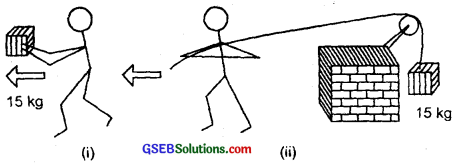(a) The heat energy required for burning of the casing of a rocket in flight is obtained from the rocket itself. The energy is obtained at the expense of the mass of the rocket and its kinetic and potential energy.
(b) The gravitational force on the comet due to Sun is a conservative force. Since the work done by a conservative force over a closed path is always zero (irrespective of the nature of path). Hence the work done by the gravitational force over every complete orbit of the comet is zero.

Aliter:
For a conservative force, work done over a path is minus of change in P.E. over a complete orbit, there is no change in P.E., so work done is zero.

(c) As the satellite comes closer and closer to Earth, its potential energy decreases. Since according to the law of conservation of energy, the sum of kinetic and potential energy must remain constant, so the kinetic energy and hence velocity of the satellite increases. However, the total energy of the satellite continuously decreases some-what due to the loss of energy against friction i.e. atmospheric resistance.

(d) In the case shown in fig. (i), the man applies the force on the mass 15 kg in vertically upward direction but walks 2m in the horizontal direction so the angle between F and S is 90°, hence work done W = F.S is zero. In fig. (ii), the force applied on the mass of 15 kg in the vertically upward direction (= wt of the body) and the mass is also lifted along the vertical so θ = 0
∴ W = mgS
= 15 × 9.8 × 2
= 294 J
Thus work done in second case is greater.

Question 6.
Underline the correct alternative:
(a) When a conservative force does positive work on a body, the potential energy of the body increases/decreases/remain unaltered.
(b) Work done by a body against friction always results in a loss of its kinetic/potential energy.
(c) The rate of change of total momentum of a many particle system is proportional to the external force/sum of the internal forces on the system.
(d) In an inelastic collision of two bodies, the quantities which don’t change after the collision are the total kinetic energy/ total linear momentum/total energy of the system of two bodies.
(a) Potential energy of the body decreases. The conservative force does positive work on a body, when it displaces the body in the direction of the force. The body therefore approaches the centre of force thus, decreasing x. Hence P.E. decreases.

(b) Work is done by a body against friction at the expense of its kinetic energy, hence work done by a body against friction results in a loss of its kinetic energy.

(c) Internal forces cannot change the total or net momentum of a system. Hence the rate of change of total momentum of many particle sy stem is proportional to the external force on the system.

(d) In an elastic collision of two bodies the quantities which do not change after the collision are total linear momentum and total energy of the system of two bodies (if the system is isolated). The total K.E. of the system, is not conserved as it may change to an equivalent amount of energy in some other form.Question 7.
State if each of the following statements are true or false? Give reasons for your answer?
(a) In an elastic collision of two bodies, the momentum and energy of each body is conserved.
(b) Total energy of a system is always conserved, no matter what internal and external forces on the body are persent.
(c) Work done in the motion of a body over a closed loop is zero for every force in nature.
(d) In an inelastic collision, the final kinetic energy is always less than the initial kinetic energy of the system.
(a) False. The total momentum and total energy of the system are conserved and not of each body.

(b) False. The external forces on the system may change (increase or decrease) the total energy of the system.

(c) False. Work done in the motion of a body over a closed loop is zero only when the body moves under the action or conservative force (like gravitational or electrostatic forces). It is not zero when the forces are non-conservative e.g. frictional forces etc.

(d) True. Usually but not always because in an inelastic collision, some kinetic energy usually changes into some other forms of energy.

Question 8.
(a) In an elastic collision of two billiard balls, is the total kinetic energy conserved during the short time of collision of the balls (i.e., when they are in contanct)?
(b) Is the total linear momentum conserved during the short time of an elastic collision of two balls?
(c) What are the answers to (a) and (b) for an inelastic collision ?
(d) Is the potential energy of two billiard balls depends only on the separation distance between their centres, is the collision elastic or inelastic? (Note, we are talking here of potential energy corresponding to the force during collision, not gravitational potential energy).
(a) No, the total kinetic energy is not conserved during the given elastic collision because a part of the kinetic energy is used in deforming the balls in that short interval for which they are in contact during collision and gets converted into potential energy. In an elastic collision, the K.E. before and after collision is same.

(b) Yes, the total linear momentum is conserved during the short time of an elastic collision of two balls.

(c) In an inelastic collision, total K.E. is not conserved during collision and even after collision. The total linear momentum is however conserved during as well as after collision.

(d) The collision is elastic.Question 9.
A body is initially at rest. It undergoes one-dimensional motion with constant acceleration. The power delivered to it at time t is proportional to

1. t1/2
2. t
3. t1/2
4. t2.

Let m = mass of the body
a = acceleration produced in the body
v = velocity of the body
P = Power delivered to the body in a time t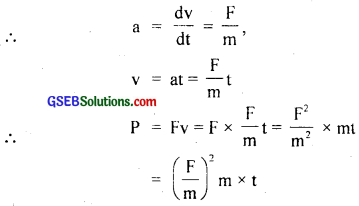or P = a2mt
Since a and m are constant; therefore
P = constant x × t
or P ∝ t,
∴ Ans. = (ii)

Question 10.
A body is moving uni-directionally under the influence of a source of constant power. Its displacement in time t is proportional to

1. t1/2
2. t
3. t3/2
4. t2

Let the constant power P acts on the body of mass m for a time t to give it a velocity v.
∴ Its K.E. = work done = power × time
or $$\frac{1}{2}$$ mv2 = Pt
or v = $$\sqrt{\frac{2 P t}{m}}=\sqrt{\frac{2 P}{m}} t^{1 / 2}$$ ………………….. (1)
Also we know that
V = $$\frac{dx}{dt}$$
or dx = vdt ……………………….. (2)
If x be the displacement of the body, then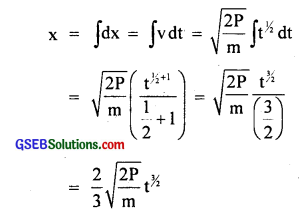Here P = constant and m is also constant for a body,
so x = constant × t1/2 or x ∝ t1/2.
∴ Ans. = (iii)

Aliter:
P = Fv = mav
Here v = u + at = 0 + at
∴ P = ma.at = ma2t
or a = $$\sqrt{P/mt}$$
Also from equation
s = ut + $$\frac{1}{2}$$ at2,
we get x = $$\frac{1}{2}$$ at2 = $$\frac{1}{2}$$ $$\sqrt{P/mt}$$ t2
= $$\frac{1}{2}$$ $$\sqrt{P/m}$$ t3/2
As P = constant (given) and m = constant
x ∝ t3/2.Question 11.
A body constrained to move along z axis of a co-ordinate system is subjected to a constant force $$\vec{F}$$ given by
F = – $$\hat {i}$$ + 2$$\hat {j}$$ + 3$$\hat {k}$$N
where $$\hat {i}$$, $$\hat {j}$$ and $$\hat {k}$$ are the unit vector along the x, y and z axis of the system respectively. What is the work done by this force in moving the body a distance of 4 m along the z axis?
Since the body is displaced 4 m along z axis only
∴ S = 4$$\hat {k}$$ = (0$$\hat {i}$$ + o$$\hat {j}$$ + 4$$\hat {k}$$)m
Also F = – $$\hat {i}$$ + 2$$\hat {j}$$ + 3$$\hat {k}$$
∴ Work done (W) is given by
W = F . S
= (- $$\hat {i}$$ + 2$$\hat {j}$$ + 3$$\hat {k}$$).(0$$\hat {i}$$ + 0$$\hat {j}$$ + 4$$\hat {k}$$)
= (-1) × o + 2 × 0 + 3 × 4
= 12 J.

Question 12.
An electron and proton are detected in a cosmic ray experiment, the first with kinetic energy 10 keV, and the second with 100 keV. Which is faster, the electron or the proton? Obtain the ratio of their speeds, (electron mass = 9.11 × 10-31 kg, proton mass = 1.67 × 10-27 kg, 1 eV = 1.60 × 10-19).
K.E. of electron = 10 kev
= 10 × 103 ev
= 104 × 1.6 × 10-19 J
or $$\frac{1}{2}$$ mev2e = 1.6 × 10-15 J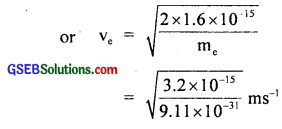(∵ me = 9.11 × 10-31 kg)
or ve = 5.93 × 107 ms-1 …………………… (i)
Mass of proton, mp = 1.67 × 10-27 kg
K.E of proton = 100 kev
= 102 × 103 × 1.6 × 10-19 J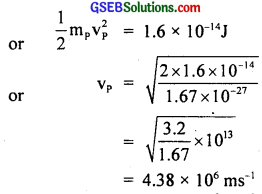= 4.38 × 106 ms-1
or vp = 4.38 × 106 ms-1 ………………….. (ii)
From (i) and (ii), we see that the speed of electron is greater than that of proton. Hence the electron moves faster than the proton.
The ratio of their speeds is given by
$$\frac{\mathbf{V}_{{e}}}{{V}_{{P}}}$$ = $$\frac{5.93 \times 10^{7}}{4.38 \times 10^{6}}$$
= 13.5.

Question 13.
A rain drop of radius 2mm falls from a height of 500m above the ground. It falls with decreasing acceleration (due to viscous resistance of the air) until at half its originial height, it attains its maximum (terminal) speed, and moves with uniform speed thereafter. What is the work done by the gravitational force on the drop in the first and second half of its journey? What is the work done by the resistive force in the entire journey if its speed oh reaching the ground is 10 ms-1?
Here, radius of the rain drop,
r = 2mm = 2 × 10-3 m
density of water drop ρ = 103 kg m-3
Height above the ground from where the drop falls, H = 500 m
Distance travelled in the half journey,
S = $$\frac{H}{2}$$ = $$\frac{500}{2}$$ = 250 m
∴ Volume of the drop, V = $$\frac{4}{3}$$ πr3
or V = $$\frac{4}{3}$$ × π × (2 × 10-3)3
= 3.35 × 10-8 m-3
∴ If m be the mass of the rain drop, then
m = Vρ = 3.35 × 10-8 × 103
= 3.35 × 10-5 kg
∴ Gravitational force on the drop,
F = mg = 3.35 × 10-5 × 9.8N
∴ Work done by the gravitational force on the drop in the first half of its journey is given by
W = FS = 3.35 × 10-5 × 9.8 × 250J
= 0.082 J
Since the distance travelled in the second half is also same, therefore, work done in the second half is also same i.e. 0.082 J
Let us assume that the rain drop is initially at rest
i.e. u = 0
Final velocity on hitting the ground
v = 10 ms-1
∴ change in K.E. of the rain drop,
∆Ek = $$\frac{1}{2}$$ mv2 – $$\frac{1}{2}$$ mu2
= $$\frac{1}{2}$$ mv2 – 0
= $$\frac{1}{2}$$ × 3.35 × 10-5 × (10)2
= $$\frac{1}{2}$$ mv2 – 0
= $$\frac{1}{2}$$ × 3.35 × 10-5 × (10)2
= 1.675 × 10-3 J = 0.00167 J
Work done by the resistive force in the entire journey
= change in K.E. – work done by gravitational force
= 0.001675 – 0.16415
= – 0.163 J.
i.e. the work done by the resistive force is negative.Question 14.
A molecule in a gas container hits a horizontal wall with speed 200 ms-1 and angle 30° with the normal and rebounds with the same speed. Is momentum conserved in the collision? Is the collision elastic or inelastic?
Yes, linear momentum is always conserved whether the collision is elastic or inelastic. Thus in the given situation, momentum is conserved.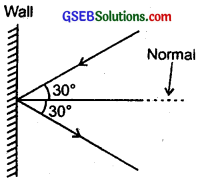As the speed of the molecule remains the same before and after the collision, so kinetic energy of the molecule is also conserved. Hence the collision is elastic.

Question 15.
A pump on the ground floor of a building can pump up water to All a tank of value 30 m3 in 15 min. If the tank is 40 m above the ground, and the efficiency of the pump is 30%, how much electric power is consumed by the pump?
Mass of water to be pumped up,
m = volume × density of water
= 30 × 103
= 3 × 104 kg
Height of the tank, h = 40 m
∴ Work done by the pump to fill the tank,
W = mgh = 3 × 104 × 9.8 × 40 J
= 1.176 × 107 J
Time, t = 15 minutes = 15 × 60s
= 900s
∴ Required power, P = $$\frac{W}{t}$$ = $$\frac{1.176 \times 10^{7}}{900}$$ = 13.07 kW
% efficiency of pump = 30%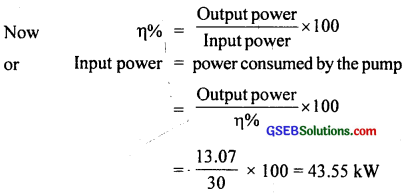= $$\frac{13.07}{30}$$ × 100 = 43.55 kW
= 43.6 kW.

Question 16.
Two identical ball bearings in contact with each other and resting on a frictionless table are hit head-on by another ball bearing of the same mass moving initially with a speed V. If the collision is elastic, which of the following is a possible result after collision?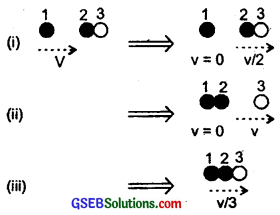Let m = mass of each ball bearing
Before collision, total K.E. of the system
= $$\frac{1}{2}$$ mv2 + 0 = $$\frac{1}{2}$$ mv2 …………………… (i)
After collision. K.E. of the system is
case (i) = $$\frac{1}{2}$$ 2m($$\frac{v}{2}$$)2 = $$\frac{1}{4}$$ mv2 ……………………… (ii)
case (ii) = $$\frac{1}{2}$$ mv2 …………………….. (iii)
case (iii) = $$\frac{1}{2}$$ (3m) ($$\frac{v}{3}$$)2 = $$\frac{1}{6}$$ mv2 ……………………. (iv)
Thus from above equation, we observe that the K.E. is conserved only in case (ii), hence case (ii) is the only possible result after collision.Question 17.
The bob A of a pendulum released from 30° to the vertical hits another bob B of the same mass at rest on a table as shown in fig. given below. How high does the bob A rise after the collision? Neglect the size of the bobs and assume the collision to be elastic?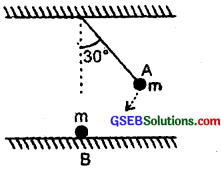We know that in perfectly elastic head on collision, when two equal masses collide with each other, then they exchange their speeds. In the present case, bob A is moving with certain speed and bob B is at rest. Hence after collision, bob A comes to rest and the bob B starts moving with the speed of the bob A. The bob A transfers whole of its momentum to ball B and hence bob A, will not rise at all after the collision.

Question 18.
The bob of a pendulum is released from a horizontal position A as shown in the figure. If the length of the pendulum is 1.5 m, what is the speed with which the bob arrives at the lower most point B, given that it dissipated 5% of its initial energy against air resistance?
At point A, the energy of the pendulum is entirely P.E. At point B, the energy of the pendulum is entirely K.E. It means that as the bob of the pendulum lowers from A to B, P.E. is converted into K.E. Thus, at B, K.E = P.E. But 5% of the P.E. is dissipated against air resistance.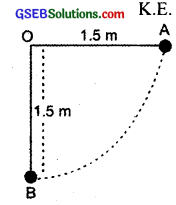K.E. at B = 95% of P.E. at A …………………… (i)
m = mass of bob
v = it speed at B,
h = height of point A w.r.t. B
= 1.5 m.
∴ from (i), $$\frac{1}{2}$$ mv2 = $$\frac{95}{100}$$ mgh
or v2 = 2 × $$\frac{95}{100}$$ gh = 2 × $$\frac{95}{100}$$ × 9.8 × 1.5
or v = $$\sqrt{\frac{19 \times 9.8 \times 1.5}{10}}$$ = $$\sqrt{27.93}$$
= 5.285 ms-1
or v = 5.3 ms-1.

Question 19.
A trolley of mass 300 kg carrying a sand bag of 25 kg is moving uniformely with a speed of 27 km/hr on a frictionless track. After a while, sand starts leaking out of a hole on the floor of trolley at the rate o.05 kg s-1. What is the speed of the trolley after the entire sand bag is empty?
The trolley carrying a sand bag is moving with a speed of 27 kmh-1. It means that the system of trolley and sand bag is not acted upon by any external force. If the sand leaks out of a hole on the floor of trolley, it does not give rise to any external force on the trolley. Therefore, speed of the trolley will not change when the sand is leaking out or even after the sand bag becomes empty. This is because the weight of sand is balanced by the normal reaction of the floor. Both of which are perpendicular to the direction of motion. Hence no work is done on the trolley or by the trolley during the leaking of the sand. So speed remains same.
∴ Speed = 27 kmh-1.Question 20.
A body of mass 0.5 kg travels in a straight line with velocity v = ax3/2, where a = 5 m-1/2s-1. What is the work done by the net force during its displacement from x = 0 to x = 2m?
Here m = 0.5 kg
v = ax3/2
a = 5 m-1/2s-1.
Let F = net force acting on the body and producing an acceleration ‘a’ in it
∴ F = ma = m. $$\frac{dv}{dt}$$
If dW be the work done by F in displacing the body by dx,
Then dW = Fdx = m$$\frac{dv}{dt}$$ dx
= m dv. $$\frac{dx}{dt}$$ = m dv(v)
= mv dv ……………….. (1)
If W be the total work done in displacing the body from x = 0 to x = 2m, then integrating equation (1), we get
W = ∫dW = m∫vdv = m $$\frac{\mathbf{v}^{2}}{2}$$
= $$\frac{1}{2}$$ m(ax3/2)2 = $$\frac{1}{2}$$ m × a2x3
Putting x = 2 – 0 = 2m, a = 5, m = 0.5, we get
W = $$\frac{1}{2}$$ × 0.5 (5)2 × (2)3
= $$\frac{1}{2}$$ × $$\frac{1}{2}$$ × 25 × 8 = 50 J.

Question 21.
The blades of a windmill sweep out a circle of area A?
(a) If the wind flows at a velocity v perpendicular to the circle, what is the mass of the air passing through it in time?
(b) What is the kinetic energy of the air?
(c) Assume that windmill converts 25% of the wind’s energy into electrical energy, and that A = 30m2, v = 36km/h and the density of air is 1.2 kg m-3. What is the electrical power produced?
(a) Here, circular area swept out by blades of windmill = A
velocity of wind = v
Let density of air = ρ
∴ Distance covered by wind in time t = vt
∴ Volume of air passing through the circular area A
= Area × length
= A vt
If m be the mass of air passing through the area A,
Then m = density × volume
= ρAvt

(b) If E be the K.E. of the air passing through area A, then
E = $$\frac{1}{2}$$ mv2
= $$\frac{1}{2}$$ ρAvt. v2 = $$\frac{1}{2}$$ ρAv3t

(c) Here A = 30 m2
v = 36 kmh-1
= 36 × $$\frac{5}{18}$$ ms-1 = 10ms-1
ρ = 1.2 kgm-3
wind’s energy = Input energy = $$\frac{1}{2}$$ ρAv3t
Electrical energy = Output energy
n% = 25 =× 100
or electrical energy = $$\frac{25}{100}$$ × $$\frac{1}{2}$$ ρAv3t
or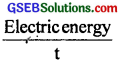= $$\frac{1}{4}$$ × $$\frac{1}{2}$$ × 1.2 × 30 × 103
= $$\frac{1}{8}$$ × $$\frac{12}{10}$$ × 30 × 1000
or Electric power produced = 4.5 × 103W = 4.5 kW.Question 22.
A person trying to loose weight (dieter) lifts a 10 kg mass 0. 5 m 1000 times. Assume that the potential energy lost each time she lowers the mass is dissipated?
(а) How much work does she do against the gravitational force?
(b) Fat supplies 3.8 × 107 J of energy per kilogram, which is converted to mechanical energy with a 20% efficiency rate. How much fat will the dieter use up?
(a) Here, m = 10 kg
h = 0.5 m
n = no. of times the mass lifted upto 0.5 m = 1000
∴ H = total height
= nh = 1000 × 0.5 m
= 500 m
work done against gravitational force = mgh = 10 × 9.8 × 500
= 49000 J

(b) Energy supplied by fat per kilogram = 3.8 × 107 J
i.e. 3.8 × 107 J energy is equivalent to 1 kg of fat
∴ 49 × 103 J energy will be equivalent to
= $$\frac{49 \times 10^{3}}{3.8 \times 10^{7}}$$ kg fat
Fat produced = $$\frac{49}{3.8}$$ × 10-4 kg
n% = 20%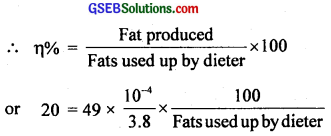or Fats used up by the dieter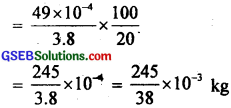= 6.447 × 10-3 kg
= 6.45 × 10-3 kg.Question 23.
A large family uses 8kW of power.
(a) Direct solar energy is incident on thd horizontal surface at an average rate of 200W per square metre. If 20% of this energy can be converted to useful electrical energy, how large an area is needed to supply 8kW?
(b) Compare this area to that of the roof of a typical house.
Here, total power used by the family = 8kW = 8000 W
(a) Average rate of incidence of the direct solar energy on the
horizontal surface = 200 W/m2.
Energy converted into useful electrical energy
= 20% of 200 W/m2
= $$\frac{20}{100}$$ × 200 = 40 W/m2
Total power (energy) required by family = 8000 W
∴ Area required to produce the same amount of electrical energy = $$\frac{8000}{40}$$ = 200 m2.

(b) In order to compare this area to that of the roof of a typical house, let ‘a’ be the side of the roof
∴ area of roof = a × a = a2
Thus a2 = 200m2
or a = $$\sqrt{200}$$ m2 = 14.14 m
∴ area of roof = 14.14 × 14.14 m2
Thus 200m2 is comparable to the roof of a large house of dimensions 14.14m × 14.14m
= 14m × 14m.s